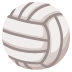# How can I make my game create a new ball and/or restart the code?

I am currently trying to make it so when a button is clicked, it will create a new ball. I’m also trying to have another button that would completely restart the game. Can someone help me out please? My code is here:

``````var gameStart = false;
x: 10,
y: 235,
w: 15,
h: 100,
};
x: 775,
y: 235,
w: 15,
h: 100,
};
var ball = {
x: 50,
y: 250,
diam: 25,
speedX: 5,
speedY: 5,
};
var speedX = 5;
var speedY = 5;
var s = "Welcome to Political Pong! Player one controls their paddle with the a and z keys. Player two controls their paddle with the /? key and the '\" key. Press the space bar to begin";
var score = 0;
var score1 = 0;
/*function down(x){
x = x + 5;
}*/

function setup() {
createCanvas(800, 600);
smooth();
//background(0);
//fill(255)
//text(s, 10, 10, 70, 80);
var button = createButton("New Ball")
button.mousePressed(createBall)
var button1 = createButton("New Game")
}

function draw() {

//if (keyPressed(32) === true) {
gameStart === true;
//}

background(0, 0, 0);
noStroke();

createBall();
ballBounceTopAndBottom();
ballBounceRight();
ballBounceLeft();
}
function createBall(){
//Create ball
fill(198, 237, 44);
ellipse(ball.x, ball.y, ball.diam, ball.diam);

ball.x = ball.x + speedX;
ball.y = ball.y + speedY;
}

fill(500, 55, 154);
if (keyIsDown(90) === true) {
}
}
if (keyIsDown(65) === true) {
}
}
}
fill(154, 55, 500);

if (keyIsDown(191) === true) { //move paddle down
}
}
if (keyIsDown(222) === true) { //move paddle up
}
}
}

function ballBounceTopAndBottom() {

//If if the ball hits the top or bottom of the court it bounces
if (ball.y + 12.5 > height || ball.y < 12.5 && ball.x > 0 && ball.x < width && ball.y > 0 && ball.y < height) {
speedY = speedY * -1; //reverse the direction of the motion
ball.y = ball.y + speedY; //keeps things moving
print("wham");

}

}
function ballBounceRight() {
//if the x of the edge ball is more than the x of the right paddle and
//the y of the ball is greater than the y of the rectangle and
//less than the y of the rectangle plus the height
if (ball.x + 12.5 > paddleR.x && ball.y + 12.5 > paddleR.y && ball.y + 12.5 < paddleR.y + paddleR.h && ball.x > 0 && ball.x < width && ball.y > 0 && ball.y < height) {
speedX = speedX * -1; //This reverses the direction, I think
ball.x = ball.x + speedX; //This keeps the ball moving
score1++;
print("bam");

}
//if the edge of the ball is lower than rect y and
//the x of the ball is greater than the x of the rect and less than the width
else if (ball.y + 12.5 > paddleR.y && ball.y < paddleR.y + paddleR.y + paddleR.h && ball.x + 12.5 > paddleR.x && ball.x < paddleR.x + paddleR.x && ball.x > 0 && ball.x < width && ball.y > 0 && ball.y < height) {
speedY = speedY * -1; //reverse the direction of the motion
ball.y = ball.y + speedY; //keeps things moving
print("bam top");
}

//if the edge of the ball is higher than rect y plus height and
//the x of the ball is greater than the x of the rect and less than the width
else if (ball.y + 12.5 < paddleR.y + paddleR.h && ball.y > paddleR.y && ball.x > paddleR.x && ball.x < paddleR.x + paddleR.h && ball.x > 0 && ball.x < width && ball.y > 0 && ball.y < height) {
speedY = speedY * -1; //reverse the direction of the motion
ball.y = ball.y + speedY; //keeps things moving
print("bam bottom");
}
}

function ballBounceLeft() {
//if the ball hits the left wall
/* if (ball.x < 0) {
speedX = speedX * -1; //This reverses the direction, I think
ball.x = ball.x + speedX; //This keeps the ball moving
print("pow");*/

//if the ball hits the front of the left paddle
if (ball.x - 12.5 < paddleL.x + paddleL.w && ball.y + 12.5 > paddleL.y && ball.y + 12.5 < paddleL.y + paddleL.h && ball.x > 0 && ball.x < width && ball.y > 0 && ball.y < height) {
speedX = speedX * -1; //This reverses the direction, I think
ball.x = ball.x + speedX; //This keeps the ball moving
score++;
print("pow");
}
fill(0, 255, 255);
textSize(24);
text("Score: " + score, 10, 25);
fill(0, 255, 255);
textSize(24);
text("Score: " + score1, 700, 25);
}
``````

I would take the time to tidy your code and fix your formatting for the forum, first … also change your `"` to `'` when defining your string and in your button arguments.

properly comment out the functions you are not using.

then create a ball object, I would suggest a class and implement vector math for physics.

to create a new ball on button press, just create a new instance of your ball object inside `createBall()`.

to start a new game, create a function that has all your initial settings and call that when button is pressed.

Hi @GoToLoop! Please provide an explanation or description in the future please. Also, the examples were removed.

Well, you’ve just butchered those 2 poor links. Gonna repost them correctly so that’s evident:1. `http://p5js.ProcessingTogether.com/sp/pad/export/ro.CxS6E9WWAMC\$EO`
2. `http://p5js.ProcessingTogether.com/sp/pad/export/ro.CwHWpJ\$SP1EN8i`

They’re about creating clickable balls. Satisfied now?oh okay, my mistake. I was trying to make the links clickable:-)

They can be turned clickable in the former forum.But in this 1, the `\$` character is butchered. And I’ve got no more ideas on how to bypass that!